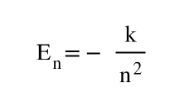# Problem: Using the Bohr model, determine the energy, in joules, necessary to ionize a ground-state hydrogen atom. Show your calculations.

###### FREE Expert Solution

The equation of energy from Bohr model states that:where En is the energy at nth level, (2.179x10-18J) is a constant and n is the number of shell

86% (185 ratings)###### Problem Details

Using the Bohr model, determine the energy, in joules, necessary to ionize a ground-state hydrogen atom. Show your calculations.